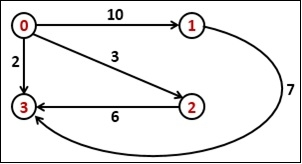# Shortest path with exactly k Edges

Data StructureGraph AlgorithmsAlgorithms

One directed graph is provided with the weight between each pair of vertices, and two vertices u and v are also provided. Our task is to find the shortest distance from vertex u to vertex v, with exactly k number of edges.To solve this problem, we will start from vertex u and go to all adjacent vertices and recur for adjacent vertices using the k value as k - 1.

## Input and Output

Input:
The cost matrix of the graph.
0 10 3 2
∞  0 ∞ 7
∞  ∞ 0 6
∞  ∞ ∞ 0

Output:
Weight of the shortest path is 9

## Algorithm

shortKEdgePath(u, v, edge)

Input − Vertex u and v, and a number of edges.

Output − Distance of shortest path.

Begin
if edge = 0 and u = v, then
return 0
if edge = 1 and cost[u, v] ≠ ∞, then
return cost[u, v]
if edge <= 0, then
return ∞
set shortPath := ∞

for all vertices i, do
if cost[u, i] ≠ ∞ and u ≠ i and v ≠ i, then
tempRes := shortKEdgePath(i, v, edge - 1)
if tempRes ≠ ∞, then
shortPath = minimum of shortPath and (cost[u,i]+tempRes
done
return shortPath
End

## Example

#include <iostream>
#define NODE 4
#define INF INT_MAX
using namespace std;

int cost[NODE][NODE] = {
{0, 10, 3, 2},
{INF, 0, INF, 7},
{INF, INF, 0, 6},
{INF, INF, INF, 0}
};

int minimum(int a, int b) {
return (a<b)?a:b;
}

int shortKEdgePath(int u, int v, int edge) {
if (edge == 0 && u == v)    //when 0 edge, no path is present
return 0;
if (edge == 1 && cost[u][v] != INF)    //when only one edge, and (u,v) is valid
return cost[u][v];
if (edge <= 0)    //when edge is -ve, there are infinity solution
return INF;
int shortPath = INF;

for (int i = 0; i < NODE; i++) {    //for all vertices i, adjacent to u
if (cost[u][i] != INF && u != i && v != i) {
int tempRes = shortKEdgePath(i, v, edge-1);
if (tempRes != INF)
shortPath = minimum(shortPath, cost[u][i] + tempRes);
}
}
return shortPath;
}

int main() {
int src = 0, dest = 3, k = 2;
cout << "Weight of the shortest path is " << shortKEdgePath(src, dest, k);
}

## Output

Weight of the shortest path is 9